1596286260

# Decision Tree -Classification

Decision Tree is one of the most widely used machine learning algorithm. It is a supervised learning algorithm that can perform both classification and regression operations.

As the name suggest, it uses a tree like structure to make decisions on the given dataset. Each internal node of the tree represent a “decision” taken by the model based on any of our attributes. From this decision, we can seperate classes or predict values.

Let’s look at both classification and regression operations one by one.

# Classification

In Classification, each leaf node of our decision tree represents a **class **based on the decisions we make on attributes at internal nodes.

To understand it more properly let us look at an example. I have used the Iris Flower Dataset from sklearn library. You can refer the complete code on Github — Here.

Image for post

A node’s samples attribute counts how many training instances it applies to. For example, 100 training instances have a petal width ≤ 2.45 cm .

A node’s value attribute tells you how many training instances of each class this node applies to. For example, the bottom-right node applies to 0 Iris-Setosa, 0 Iris- Versicolor, and 43 Iris-Virginica.

And a node’s gini attribute measures its impurity: a node is “pure” (gini=0) if all training instances it applies to belong to the same class. For example, since the depth-1 left node applies only to Iris-Setosa training instances, it is pure and its gini score is 0.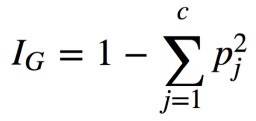Gini Impurity Formula

where, pⱼ is the ratio of instances of class j among all training instances at that node.

Based on the decisions made at each internal node, we can sketch decision boundaries to visualize the model.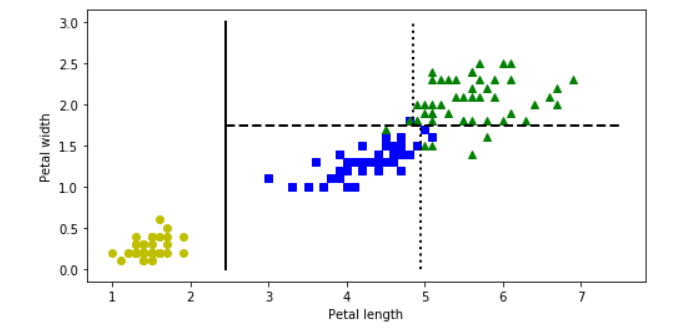But how do we find these boundaries ?

We use Classification And Regression Tree (CART) to find these boundaries.

CART is a simple algorithm that finds an attribute _k _and a threshold _t_ₖat which we get a purest subset. Purest subset means that either of the subsets contain maximum proportion of one particular class. For example, left node at depth-2 has maximum proportion of Iris-Versicolor class i.e 49 of 54. In the _CART cost function, _we split the training set in such a way that we get minimum gini impurity.The CART cost function is given as: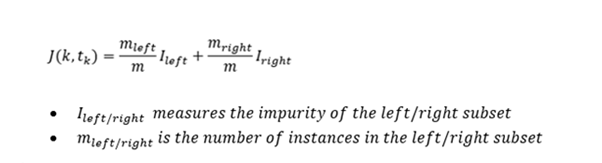After successfully splitting the dataset into two, we repeat the process on either sides of the tree.

We can directly implement Decision tree with the help of Scikit learn library. It has a class called DecisionTreeClassifier which trains the model for us directly and we can adjust the hyperparameters as per our requirements.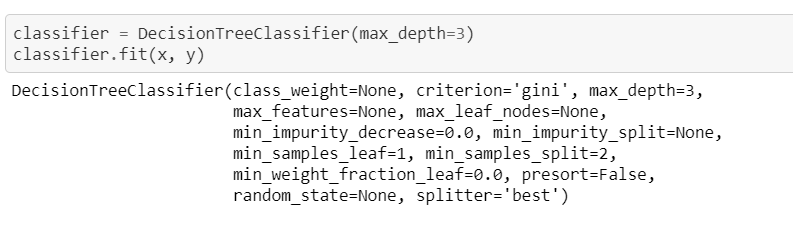#machine-learning #decision-tree #decision-tree-classifier #decision-tree-regressor #deep learning

## Buddha Community1592847556

## Binary Decision Trees

Binary Decision Trees. Binary Decision Trees
Binary decision trees is a supervised machine-learning technique operates by subjecting attributes to a series of binary (yes/no) decisions. Each decision leads to one of two possibilities. Each decision leads to another decision or it leads to prediction.

#decision-tree-regressor #decision-tree #artificial-intelligence #mls #machine-learning #programming1602997200

## Decision Tree Intuition

Decision Trees are easy & Simple to implement & interpreted. Decision Tree is a diagram (flow) that is used to predict the course of action or a probability. Each branch of the decision tree represents an outcome or decision or a reaction. Decision Trees can be implemented in a variety of situations from personal to complex situations. The sequence of steps will give a better understanding easily.

In Programming, we regularly use If-else conditions, even the Decision Tree working process is similar to an If-else condition.

## Let’s see how a decision Tree Looks like

The below tree shows a simple implementation of different nodes in the decision tree.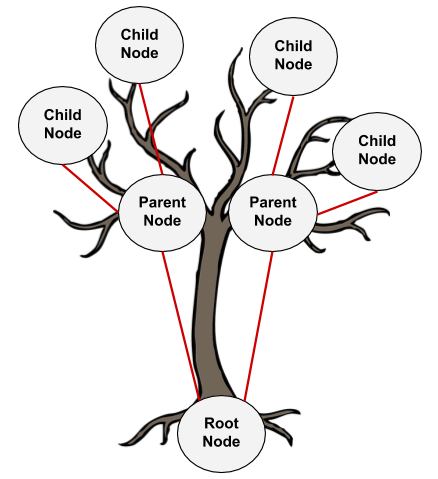1. **Root Node: **Root Node is a top node with the base feature.
2. **Parent Node: **Nodes that get their origin from a root node or this can be represented as a decision node where the decision of Yes/No or True/False or prediction turn happens.
3. **Child Node: **these nodes get their origin from a parent node. If the decision made from a parent node is not satisfactory then these nodes will be created. Until we arrive at the final node where we have pure domination in a class (Yes/No) means that until we arrive at the leaf node.
4. **Leaf Node: **Can also be called a terminal Node or a final decision node where we will conclude.

#gini-index #entropy #decision-tree #decision-tree-classifier #machine-learning1598063220

## Maths behind Decision Tree Classifier

Before we see the python implementation of the decision tree. Let’s first understand the math behind the decision tree classification. We will see how all the above-mentioned terms are used for splitting.

We will use a simple dataset which contains information about students from different classes and gender and see whether they stay in the school’s hostel or not.

This is how our data set looks like :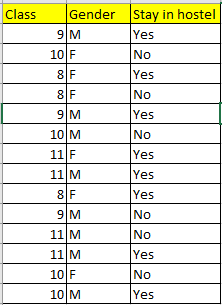Let’s try and understand how the root node is selected by calcualting gini impurity. We will use the above mentioned data.

We have two features which we can use for nodes: “Class” and “Gender”. We will calculate gini impurity for each of the features and then select that feature which has least gini impurity.

Let’s review the formula for calculating ginni impurity: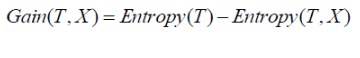Let’s start with class, we will try to gini impurity for all different values in “class”.

#data-science #machine-learning #decision-tree-classifier #decision-tree #deep learning1598227320

## Remove all leaf nodes from a Generic Tree or N-ary Tree

Given a Generic tree, the task is to delete the leaf nodes from the tree.

** Examples:**

``````Input:
5
/  /  \  \
1   2   3   8
/   / \   \
15  4   5   6

Output:
5 : 1 2 3
1 :
2 :
3 :

Explanation:
Deleted leafs are:
8, 15, 4, 5, 6

Input:
8
/    |    \
9      7       2
/ | \    |    / / | \ \
4  5 6    10  11 1 2  2 3
Output:
8: 9 7 2
9:
7:
2:
``````

**Approach: **Follow the steps given below to solve the problem

• Take tree into the vector.
• Traverse the tree and check the condition:
• If current node is leaf then
• Delete the leaf from vector
• Else
• Recursively call for every child.

Below is the implementation of the above approach:

#data structures #recursion #tree #n-ary-tree #tree-traversal #data analysis1618197121

## Learning Decision Trees - Machine Learning

In the context of supervised learning, a decision tree is a tree for predicting the output for a given input. We start from the root of the tree and ask a particular question about the input. Depending on the answer, we go down to one or another of its children. The child we visit is the root of another tree. So we repeat the process, i.e. ask another question here. Eventually, we reach a leaf, i.e. a node with no children. This node contains the final answer which we output and stop.

#ai & machine learning #decision trees #machine learning #regression tree #supervised learning